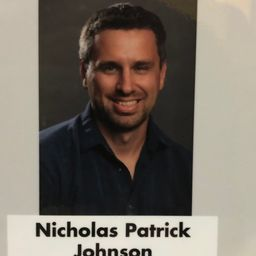# Nick J.

University of Wisconsin - Milwaukee

## Biography

Many people might say that I am a Math Enthusiast, but that is quite a bit of an understatement. I am honored to be an ambassador of The Global Math Project headed by James Tanton. James writes the forward in the beautiful book Pi of Life: The Hidden Happiness of Mathematics and says, “Mathematics is the portal to the playground of the soul.” I agree with him so much and hope to be able to show “kids” of all ages how amazing mathematics can be. To quote the author of A Mathematician’s Lament Paul Lockhart, “The only thing I am interested in using mathematics for is to have a good time and help others do the same.”

## Education

MS Mathematics
University of Wisconsin - Milwaukee
BS Education
University of Wisconsin - Milwaukee

## Topics Covered

Integrals
Integration
Applications of Integration
Derivatives
Differentiation
Applications of the Derivative
Equations and Inequalities
Whole which of Numbers
The Integers
The Language of Algebra
Fractions and Mixed Numbers
Decimals
Linear Functions
Rational Functions
Algebra Topics That are Reviewed at the Start of the Semester
Exponential and Logarithmic Functions
Functions and Their Graphs
Functions
Polynomials
Write Linear Equations
Linear Equations and Functions
Sampling and Data
Descriptive Statistics
Introduction to Sequences and Series
Introduction to Combinatorics and Probability
Graphs and Statistics
Systems of Equations and Inequalities
Introduction to Matrices
An Introduction to Geometry
Introduction to Conic Section
Limits
Continuous Functions
Vectors
Introduction to Vectors
Power Series
Partial Derivatives
Introduction to Algebra
Multiple Integrals
Sequences
Series
Ratio, Proportion, and Measurement
Trigonometry
Taylor Series
Integration Techniques
Differential Equations
Functions
Trig Integrals
Trig Substitution
Variables and Exponents
Exponents and Polynomials
Introduction to Sequences and Series
Introduction to Combinatorics and Probability
Parametric Equations
Polar Coordinates
Introduction to Conic Sections
Solve Linear Inequalities
Graph Linear Functions
Introduction to Trigonometry
Applications of Trigonometric Functions
Graphing Trigonometry Functions
Improper Integrals
Vector Functions
Complex Numbers
Right Triangles
Circles
Congruent Triangles
Relationships Within Triangles
Vector Calculus
Distribution and One-Way ANOVA
Polygons
Surface Area
Angles
Parallel and Perpendicular lines
Logic
Similarity
Matrices
Geometry Basics
Geometric Proof
Area and Perimeter
Volume
Probability
Foundations for Geometry
Transformations
Functions of Several Variables
Series Tests
Volume
1 2 3 4 5 ... 1165